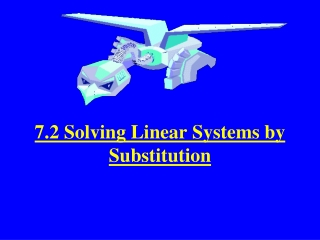DownloadDownload Presentation7.2 Solving Linear Systems by Substitution

# 7.2 Solving Linear Systems by Substitution

Download Presentation## 7.2 Solving Linear Systems by Substitution

- - - - - - - - - - - - - - - - - - - - - - - - - - - E N D - - - - - - - - - - - - - - - - - - - - - - - - - - -
##### Presentation Transcript

1. 7.2 Solving Linear Systems by Substitution

2. Steps: • 1. Solve one of the equations for one of the variables. • Substitute that expression into the other equation and solve for the other variable. This gives you the first part of your ordered pair. • Substitute this value into the revised first equation and solve. This gives you the second part of your ordered pair. • Check the solution pair in each of the original equations. If it works, you have the solution.

3. Example: -x + y = 1 2x + y = -2 • Rewrite: -x + y = 1 • y = x + 1 (you could write x = y-1) • Substitute: 2x + y = -2 • 2x + (x + 1) = -2 • 2x + x + 1 = -2 • 3x + 1 = -2 • 3x = -3 • x = -1

4. Substitute: y = x + 1 • y = -1 + 1 • y = 0 • Solution (-1, 0) • Check: -x + y = 1 2x + y = -2 • -(-1) + 0 = 1 2(-1) + 0 = -2 • 1 = 1 -2 = -2 Both are true so the solution is (-1,0). Graph to check.

5. Graph to find the solution • 2x = 5 • x + y = 1 Can you tell what the solution is? Now solve using substitution.

6. Change the second equation by solving for x Now where you have an x in the first equation, substitute in –y +1 and solve for y. x = -y + 1 You get y = -3/2 Now plug -3/2 into the second equation and solve for x. You find that x = 5/2. Solution (5/2, -3/2) Check each equation to make sure you have the right answer.

7. Real world example: Dinner at a China Buffet Adult cost is \$11.95 Children cost \$6.95 Total bill is \$61.70 Total number of people is 6 How many adults and how many children went? Write 2 equations 11.95A + 6.95C = 61.70 A + C = 6 (rewrite A = 6 – C) Substitute

8. 11.95 ( 6 - C) + 6.95 C = 61.70 (substitute) 71.70 – 11.95 C + 6.95 C = 61.70 71.70 – 5 C = 61.70 - 5C = -10 C = 2 A + C = 6 A + 2 = 6 A = 4 There are 4 adults and 2 children. 4(11.95) + 2(6.95) = 61.70 47.80 + 13.90 = 61.70 61.70 = 61.70 (Check)